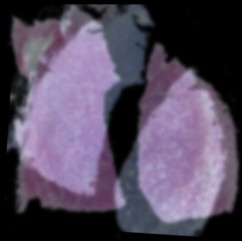Image registration is the task of finding a transformation that best aligns two given images. A typical problem could be that we have two images of a patient taken at different points in time, and the patient has moved in between.

This page demonstrates the use of rigid image registration, where one assumes that the images only have to be translated and rotated in order to align them.

Drag with the mouse or finger to move the template image. Rotate using two fingers. The algorithm will automatically try to restore the alignment.

Simple algorithms such as this one have difficulties with complicated objects: they fill only find a local minimum, which is only partially optimal, and from which the algorithm cannot escape.

Authors: Lars Koenig, Jan LellmannThe Rudin-Osher-Fatemi denoising model is an absolute classic and the prototype model for studying non-smooth regularization. The input image $I$ is split into noise and structure by minimizing the energy $$\min_{u:\Omega\to R} \int_\Omega \|u(x) – I(x)\|_2^2 dx + \lambda \int_\Omega d\|D u\|.$$
The model preserves edges in the image very well. It tends to introduce piecewise constant regions, and a great deal of research has gone into alternative regularization for reducing this effect.

Authors: Jan LellmannIn multiclass image labeling, also called multiclass image segmentation, one would like to split an image into regions based on the local image information. Usually the data is noisy, so an additional regularization term is added to make the region boundaries smooth.

The resulting minimization problem is non-smooth but also combinatorial, which makes it hard to find an exact solution. An approach for finding a good approximate solution is convex relaxation. The idea is to approximate the energy by a convex energy, of which a global minimizer can be found.

In this approach, the relaxed energy for $L$ different labels has the form
$$f(u) = \int_\Omega \langle u(x),s(x)\rangle \mathrm{d}x + \lambda \mathrm{TV}(u),$$
where every $u(x)$ is a point on the $L$-dimensional unit simplex, i.e., it is a probability measure on the labels $\{1,\ldots,L\}$.

Authors: Benedikt Löwenhauser, Kevin Schober, Jan Lellmann